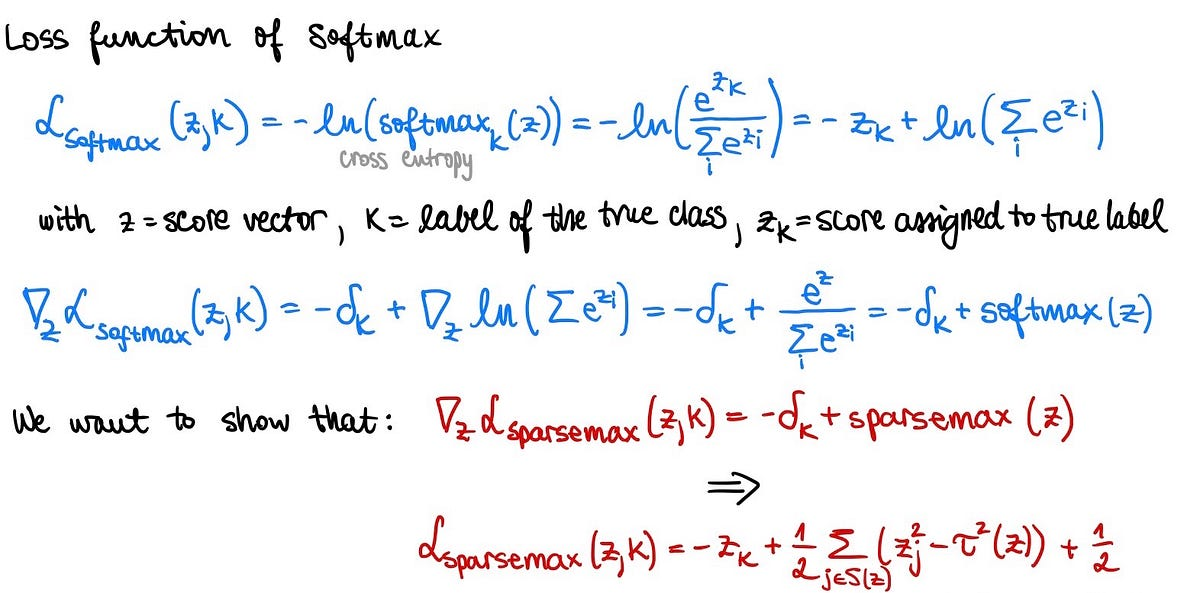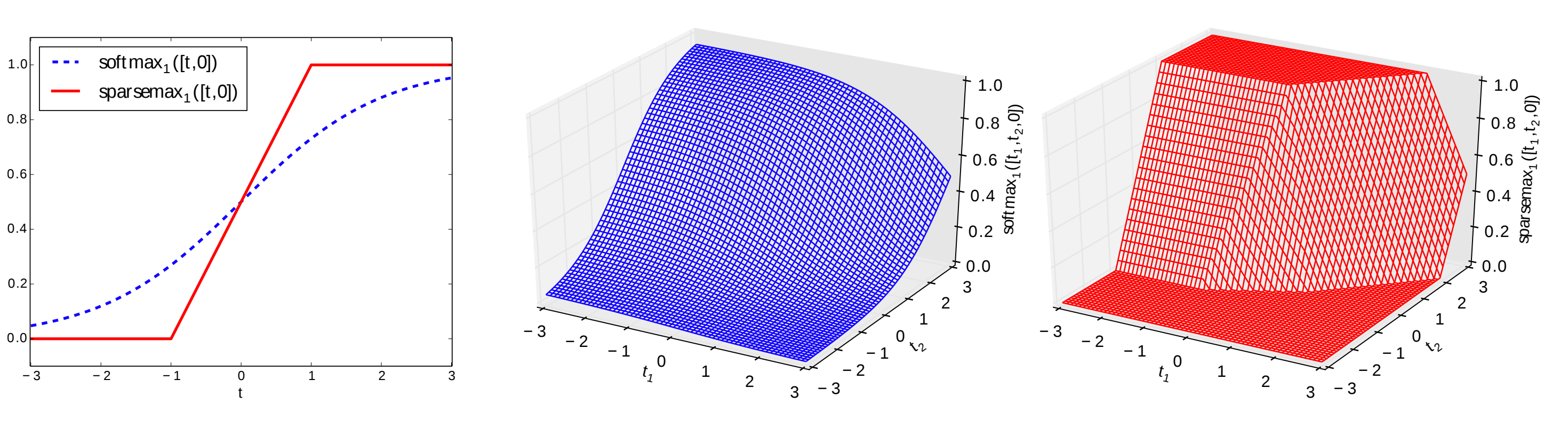# Activation Functions in Deep Learning: From Softmax to SparsemaxComplete mathematical derivation of Sparsemax activation function: Softmax alternative for sparse outputs. The objective of this post is three-fold. The first part discusses the motivation behind sparsemax and its relation to softmax, summary of the original research paper in which this activation function was first introduced, and an overview of advantages from using sparsemax.

The objective of this post is three-fold. The first part discusses the motivation behind sparsemax and its relation to softmax, summary of the original research paper in which this activation function was first introduced, and an overview of advantages from using sparsemax. Part two and three are dedicated to the mathematical derivations, concretely finding a closed-form solution as well as an appropriate loss function.

## 1 . Overview of Sparsemax

In the paper “From Softmax to Sparsemax: A Sparse Model of Attention and Multi-Label Classification”, Martins et al. propose a new alternative to the widely known softmax activation function by introducing Sparsemax.

While softmax is an appropriate choice for multi-class classification that outputs a normalized probability distribution over K probabilities, in many tasks, we want to obtain an output that is more sparse. Martins et al. introduce a new activation function, called sparsemax, that outputs sparse probabilities of a multinomial distribution and, therefore, filters out noise from the mass of the distribution. This means that sparsemax would assign a probability of exactly 0 for some classes, while softmax would instead keep those classes and assign them very small values like 10⁻³. Sparsemax can be especially favorable in large classification problems; for instance in Natural Language Processing (NLP) tasks, where the softmax layer is modeling a multinomial distribution over a very large vocabulary set.

In practice, however, changing the softmax function into a sparse estimator is not a straightforward task. Obtaining such a transformation while preserving some of the fundamental properties of softmax — e.g. simple to evaluate, inexpensive to differentiate and easily transformed to a convex loss function — turns out to be quite challenging. A traditional way around it in machine learning is to use the L1 penalty that allows for some level of sparsity with regards to the input variables and/or deep layers in neural networks. While this approach is relatively straightforward, L1 penalty influences the weights of a neural network rather than the targeted outputs as sparse probabilities. Therefore, Martins et al. recognize the need for a supplementary activation function, i.e. sparsemax, which they formulate as a solvable quadratic problem and find a solution under a set of constraints to get similar properties to softmax.

Before diving into the proofs behind sparsemax implementation, let us first discuss few important high-level findings from the paper. The following bullet points summarize some of the main takeaways:

• Sparsemax is a piecewise linear activation function

While softmax shape is equivalent to the traditional sigmoid, sparsemax is a “hard” sigmoid in one dimension. Additionally, in two dimensions, sparsemax is a piecewise linear function with entire saturated zones (0 or 1). Here is a figure from the paper to help you visualize softmax and sparsemax.## Deep Learning 101 —  Neural Networks Explained

The past few decades have witnessed a massive boom in the penetration as well as the power of computation, and amidst this information.

## Explain Deep Learning Neural Networks to your grandma

“You do not really understand something unless you can explain it to your grandmother” Not sure where this quote originally came from, it is sometimes kind of half-attributed to Albert Einstein.

## Deep Learning Explained in Layman's Terms

Deep Learning Explained in Layman's Terms. In this post, you will get to learn deep learning through a simple explanation (layman terms) and examples.

## GPT-3, a Giant Step for Deep Learning And NLP

Can intelligence emerge simply by training a big enough language model using lots of data? OpenAI tries to do so, using 175 billion parameters.

## Alibaba’s Mobile Neural Network: A deep learning framework for mobile and embedded devices

A peek at Alibaba’s Mobile Neural Network (MNN) and how it achieves balance between high performance, flexibility, and ease-of-use.